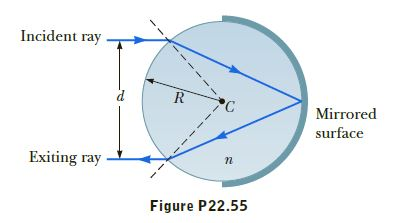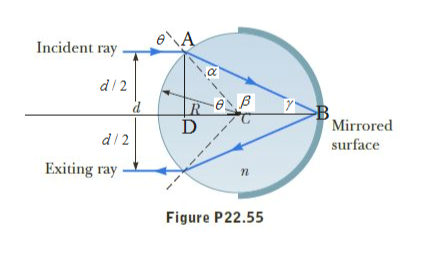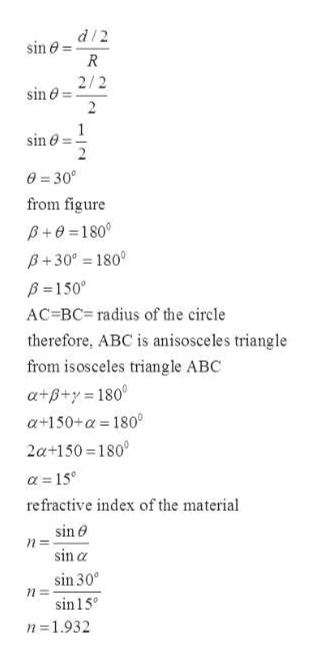Question
1 views

A transparent cylinder of radius R = 2.00 m has a mirrored
surface on its right half, as shown in Figure P22.55. A light ray

traveling in air is incident on the left side of the cylinder. The
incident light ray and the exiting light ray are parallel, and
d = 2.00 m. Determine the index of refraction of the material.help_outlineImage TranscriptioncloseIncident ray R. Mirrored surface Exiting ray Figure P22.55 fullscreen
check_circle

Step 1

Given:

distance between rays = 2m

angle of incidence = θ

angle of refraction = αhelp_outlineImage TranscriptioncloseIncident ray d/ 2 >B Mirrored d/ 2 surface Exiting ray - п Figure P22.55 fullscreen
Step 2

from triangl...help_outlineImage Transcriptionclosed/2 sin e = 2/2 sin e 2 sin o 0 = 30° from figure B+0 =180° B+30° = 180° B =150° AC=BC= radius of the circle therefore, ABC is anisosceles triangle from isosceles triangle ABC a+B+y = 180° a+150+a = 180° 2a+150 = 180° a = 15° refractive index of the material sin e sin a sin 30° sin15° n=1.932 fullscreen

### Want to see the full answer?

See Solution

#### Want to see this answer and more?

Solutions are written by subject experts who are available 24/7. Questions are typically answered within 1 hour.*

See Solution
*Response times may vary by subject and question.
Tagged in
SciencePhysics

### Other